Published: 18 August 2023

# Intelligent CNC control with improved adaptive thermal error compensation model

Hui Shen1
Liu Yang2
1, 2School of Electronics and Information, Mianyang Polytechnic, Mianyang, China
Corresponding Author:
Hui Shen
Views 64

#### Abstract

The thermal errors (TE) of computer numerical control (CNC) in workshop production seriously reduces the productivity. Therefore, to improve the productivity of CNC and enhance production intelligence, an improved adaptive TE compensation model (TECM) is proposed. The model is based on an online temperature measurement system (OTMS), which improves the adaptive learning rate of back-propagation neural network (BPNN) for temperature prediction. Finally, the correlation value between temperature changes and TEs is used for thermal error compensation. The performance verification of the model shows that the proposed OTMS can achieve effective temperature acquisition and processing, and the prediction ability of the improved BPNN is significantly higher than that of other prediction algorithms. Finally, it is found in the test that the improved adaptive TECM can reduce the contour error of workpiece machining to within 0.02×10-3 mm, the machining accuracy of CNC is significantly improved. The above results show that using the improved adaptive TECM can promote the intelligent development of CNC and improve their machining accuracy, which is of great significance to the development of workshop manufacturing.

## 1. Introduction

Computer numerical control (CNC) have gradually become an important equipment for workshop processing, because CNC can achieve intelligent control and improve productivity. However, CNC still have the same problem as traditional CNC, that is, with the increase of running time, machining errors will gradually occur [1-2]. A large number of studies that the errors of CNC include geometric errors and thermal errors (TE), among which TE are the reduction of processing accuracy caused by the temperature rise of CNC after a long time of operation, and the temperature change of CNC is difficult to control. Therefore, how to effectively solve the TE has become the key research direction to improve the airport processing accuracy . The existence of TE is caused by the temperature rise in the operation of CNC. Therefore, some studies have proposed that the TE compensation of CNC can be realized through temperature prediction. However, after a long time of discussion, the prediction methods given by a large number of studies are not ideal . In this study, an online temperature measurement system (OTMS) was designed and a back-propagation neural network (BPNN) with improved adaptive learning rate was used as the thermal deformation compensation model for temperature prediction. An adaptive thermal error compensation model was constructed to achieve intelligent machine tool control and improve the efficiency and accuracy of CNC machine tool workpiece processing.

## 2. Related works

CNC are important equipment in work piece processing. With the development of intelligent technology, CNC also show a trend of continuous optimization. To improve the productivity of production and manufacturing, Aktas et al.  put forward a selection program considering the influence of different factors on CNC. This program can select the best CNC router of CNC by analyzing the uncertainty and variability of statements. The proposed method can improve the CNC effect of CNC in complex environments. Ye et al.  believed that it was necessary to improve the intelligent level of CNC, and proposed a CNC controller to realize the process planning of CNC with the support of cloud database. The method proposed in this study is feasible and can effectively improve the working efficiency of CNC. Cheng et al.  proposed a standard for the exchange of product model date-network computer (STEP-NC) method for the energy consumption of CNC, and used this method to build the energy consumption calculation model. STEP-NC method has fast estimation efficiency and can improve the intelligent level of CNC. Jeon et al.  put forward a new era oriented CNC system, which takes into account multiple interests and aims to improve the intelligent level of industrial production. The application test shows that the CNC system proposed in the study has a good application effect, and has an obvious level of intelligence. Martinova et al.  proposed a monitoring system for evaluating CNC, which evaluates their health by analyzing the life cycle of CNC. The monitoring system proposed in the study can effectively check the performance of CNC to ensure their normal operation.

In the work of CNC, most CNC will produce errors due to various factors. A large number of scholars have put forward their own views on error handling of CNC. Liu et al.  believed that the time-varying errors of CNC during operation had a great impact on their machining accuracy, so they proposed a time-varying error compensation model, which introduced digital twins to predict and compensate the errors. The proposed method can effectively reduce the error, and then improve the machining accuracy. Mechta et al.  put forward an evaluation method for the axis error of CNC, and evaluated the effectiveness of the error evaluation method through comparative analysis. The error evaluation method proposed in the study has high accuracy and can accurately predict the axis error of CNC. Liu et al.  proposed a method to identify dynamic error and static error, which can reflect the geometric error in the work of CNC. The result analysis shows that the method proposed in the study can practice a variety of error identification and separation, and its measurement efficiency is significantly higher than that of traditional measurement methods, proving that the method is feasible and efficient. Liu et al.  proposed a dynamic linear model of TE to avoid the change of machining accuracy caused by TE of CNC. This model is used to compensate TE, thus improving the stability of CNC processing. The proposed model can improve the stability of CNCs and the accuracy of work piece processing. Katageri et al.  believed that the machining accuracy of CNC was affected by the stability of the CNC itself, and believed that the TE of the CNC would significantly affect the machining accuracy. Therefore, they studied and analyzed the identification ability of different methods in the thermal sensitive area, and proposed a TE compensation method based on this.

To sum up, to improve the productivity of the manufacturing industry, the intelligence of CNC is crucial. To improve the processing accuracy of CNC, a large number of scholars have proposed error analysis and compensation methods. However, it can be seen from the current research status that TE has a significant impact on the accuracy of CNC. To reduce the influence of TE on CNC, a TECM is proposed to promote the intelligent development of CNC.

## 3. Improved adaptive TECM design of CNC

### 3.1. On line measurement system for TE of CNC

In the process of TE compensation of CNCs, it is necessary to measure the TE of CNC to build a measurement system. In the current CNC work, most TE measurement systems use sensors to measure the TE of the CNC, and most sensors are fixed on the CNC, which is difficult to measure the TE of the CNC in the processing state [15-16]. Therefore, based on the machining state of the CNC, an OTMS is proposed, which uses online monitoring technology to measure the idling and working TE of the CNC. The OTMS of CNC is shown in Fig. 1.

In Fig. 1, the online measurement system is based on traditional measurement systems, including two modules: temperature sensor and position measurement. Temperature collection collects data through temperature collection cards and builds a temperature database for CNC machine tools, which are then stored in a computer. The position measurement module uses coordinate acquisition cards to detect the coordinate values during the startup, idle operation, and workpiece processing of CNC machine tools, and imports the collected coordinate values into the computer to construct a coordinate dataset. In the process of recording the coordinate value of the work piece, to ensure the accuracy of the temperature measurement of the measurement system, Tunable Diode Laser Absorption Spectroscopy (TDLAS) technology is introduced. TDLAS technology uses the two-wire technology in the temperature measurement, which can eliminate the influence of the environmental optical path, thus ensuring the measurement accuracy of the sensor [17-18]. TDLAS technology uses laser sensors to analyze target temperature changes in the measurement process. It studies the detection target of water molecules in the temperature changes of CNC, and evaluates the temperature changes by analyzing molecular spectra [19-20]. First, the spectral absorptivity ${\alpha }_{v}$ is defined in Eq. (1):

1
${\alpha }_{v}={k}_{v}l,$

where, ${k}_{v}$ represents the spectral absorption coefficient; $l$ represents the total range of the absorption path. After calculating the spectral absorption, define the integral absorption, as shown in Eq. (2):

2
$C=\int {\alpha }_{v}{d}_{v}=P\cdot M\cdot S\left(T\right)\cdot l,$

where, $P$ represents the total pressure of water molecule, in atm; $M$ is the molar concentration, in mol/L; $S\left(T\right)$ represents the spectral line intensity; $T$ is the temperature.

Fig. 1On line temperature measurement system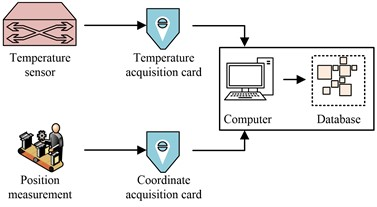In CNC temperature measurement, data acquisition and processing are more important. Data deviation caused by environmental impact can be avoided through data processing. To effectively eliminate the impact of other factors, the research proposes to use mean filtering to achieve data noise reduction . The principle of mean filtering is shown in Eq. (3):

3
$y\left(n\right)=\frac{1}{M}\sum _{i=n-j}^{n+j}y\left(i\right),$

where, $y\left(n\right)$ is the filtered result; $y\left(i\right)$ represents data to be filtered; ${S}_{mf}$ is the size of the filtering window. See Eq. (4) for the size of the filtering window:

4
${S}_{mf}=2j+1.$

In addition, the temperature information of CNC in operation has obvious time domain characteristics, including mean, variance, etc. The calculation of the mean $\stackrel{-}{X}$ is shown in Eq. (5):

5
$\stackrel{-}{X}=\frac{1}{n}\sum _{i=1}^{n}{X}_{i},$

where, $n$ represents the number of data and ${X}_{i}$ represents the th data. The calculation of standard deviation $\sigma$ is shown in Eq. (6):

6
$\sigma =\sqrt{\frac{1}{n}\sum _{i=1}^{n}{\left({X}_{i}-{\stackrel{-}{X}}_{i}\right)}^{2}}.$

The calculation of kurtosis $Kurt\left[x\right]$ of sample $x$ is shown in Eq. (7):

7
$Kurt\left[x\right]=\frac{E\left[{\left(x-\stackrel{-}{x}\right)}^{4}\right]}{E{\left[{\left(x-\stackrel{-}{x}\right)}^{2}\right]}^{2}},$

where, $x$ is the mean of the sample. $E\left(\cdot \right)$ is the mean operation. The variance is calculated according to Eq. (8):

8
${\sigma }^{2}=\frac{1}{n}\sum _{i=1}^{n}{\left({X}_{i}-\stackrel{-}{X}\right)}^{2}.$

The TE compensation of CNC is to adjust the processing by analyzing the changes of CNC temperature and processing coordinates. To further improve the accuracy of the TECM, the research first proposed the temperature prediction algorithm in the construction of the adaptive TE model [22-23]. The commonly used prediction methods currently include time series prediction, machine learning based methods, and neural network based methods. The internal structure of CNC machine tools is complex, and the temperature field has nonlinear characteristics. However, traditional methods mostly only predict short-term and medium-term aging, and when there are significant changes in the external environment, there will generally be significant deviations. Compared with traditional models, neural networks have better real-time prediction and persistence, and higher fault tolerance, which are more suitable for processing nonlinear system temperature prediction [24-25]. Therefore, the research is based on BPNN algorithm to predict the temperature information of CNC. The existing research results show that the three-layer BPNN can arbitrarily approximate any complex nonlinear system, so the three-layer BPNN is used in the temperature prediction model.

In data analysis, calculate from the input layer to realize the forward propagation of BPNN. After that, feedback is made according to the output of the output layer, and optimization by analyzing the error of the output value, so as to realize the back propagation of the BPNN. The activation function in the BPNN plays an important role in predicting the output results of the network and in the backward adjustment of errors. The sigmoid function can convert any input real number into the [0, 1] distribution space, and the function has very good symmetry, so the activation function uses sigmoid, as shown in Eq. (9):

9
$f\left(x\right)=\frac{1}{1+{e}^{-x}},$

where, $e$ represents the natural constant; $x$ represents the function value of the input. Use Eq. (10) to calculate hidden layer nodes:

10
$N=\sqrt{x+z}+y,$

where, $N$ represents the optimal number of hidden layer nodes; $x$ and $y$ respectively represent the number of neuron nodes in the input layer and output layer. $z$ is a positive integer within 1-10.

The traditional BPNN has the disadvantage of slow convergence in the calculation process. Therefore, to improve the convergence speed of the BPNN, it is proposed to use the learning rate training to improve the BPNN, as shown in Eq. (11):

11
$\eta \left(k\right)=\left\{\begin{array}{l}{\alpha }_{1}×\eta \left(k-1\right)\begin{array}{ll},& E\left(k\right)E\left(k-1\right),\end{array}\end{array}\right\$

where, $\alpha$ represents a constant; $\eta \left(k\right)$ represents the algorithm learning rate after $k$ iterations; $E\left(k\right)$ represents learning error. The calculation method of learning error is shown in Eq. (12):

12
$E\left(k\right)=\frac{\sum _{k=1}^{n}\left({y}_{k}-{\stackrel{^}{y}}_{k}{\right)}^{2}}{2},$

where, ${y}_{k}$ represents the real output; ${\stackrel{^}{y}}_{k}$ indicates predictive output. temperature prediction is realized by improving BPNN. On this basis, an adaptive TECM is built. In the compensation model, first select the temperature sensitive points and study the correlation between the bed TE and temperature variables by using the gray correlation degree, as shown in Eq. (13):

13
${\xi }_{0,i}\left(k\right)=\frac{\underset{i}{\mathrm{m}\mathrm{i}\mathrm{n}}\underset{k}{\mathrm{m}\mathrm{i}\mathrm{n}}\left|{x}_{0}\left(k\right)-{T}_{i}\left(k\right)\right|+\rho \underset{i}{\mathrm{m}\mathrm{a}\mathrm{x}}\underset{k}{\mathrm{m}\mathrm{a}\mathrm{x}}\left|{x}_{0}\left(k\right)-{T}_{i}\left(k\right)\right|}{\left|{x}_{0}\left(k\right)-{T}_{i}\left(k\right)\right|+\rho \underset{i}{\mathrm{m}\mathrm{a}\mathrm{x}}\underset{k}{\mathrm{m}\mathrm{a}\mathrm{x}}\left|{x}_{0}\left(k\right)-{T}_{i}\left(k\right)\right|},$

where, ${x}_{0}\left(k\right)$ represents the TE value of CNC; $k$ represents the total number of measuring points, and the value is $k=1,2,\cdots ,N$; Represents the $i$-th temperature measuring point; $\rho$ represents the resolution coefficient. The smaller the value, the greater the resolution. Generally, the value range of $\rho$ is [0, 1], usually 0.5.

The grey correlation analysis is used to calculate the correlation between the temperature change and the TE of CNC, and the influence of temperature on the TE is evaluated by its correlation value. In the correlation analysis, the calculated correlation values are sorted according to the size, and the first 20 temperature measuring points are selected to ensure the accuracy of prediction and facilitate the measurement of the OTMS.

Finally, the TECM is established through the construction of the OTMS and the selection of temperature measuring points. The compensation process of the TECM of CNC is shown in Fig. 2.

Fig. 2TE compensation process of CNC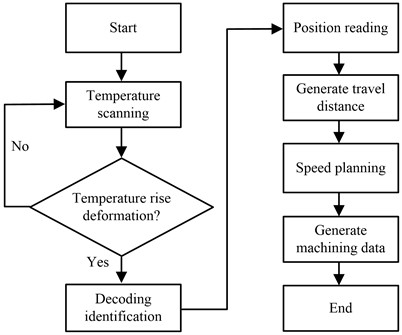Fig. 2 shows that during the TE compensation process of the CNC, first collect the temperature data of the CNC to detect whether the work piece is deformed during processing. When the work piece is detected to be deformed during processing, the current position with deviation will be read by decoding the mark again, and then error compensation will be made according to the current position and the ideal position. During error compensation, new tool travel distance will be continuously generated to correct the tool position during work piece processing. In addition, in the process of TE compensation, the processing speed of the CNC will be continuously optimized to reduce the offset at a faster speed.

### 3.3. Test and analysis of improved adaptive TECM temperature measurement effect test

A study has proposed an OTMS for CNC machine tools to address the TE. In order to verify the effectiveness of the temperature measurement system, the system was tested using the Leaderway V-450 machine tool as the experimental object. The measurement system used the OP550 online detection system developed by Harbin Xianfeng Electromechanical. The temperature acquisition system and coordinate acquisition device are designed using the STEM32 minimum system, with the temperature sensor using a magnetic adsorption type DS18B20 temperature sensor. The relevant experimental parameters are shown in Table 1.

Table 1Test parameter settings

 Step 1 Step 2 Step 3 Step 4 Simulated workingcondition 1 Spindle running (60 min) Pause (160 min) Parameter settings 1000 r/min 0 r/min Simulated workingcondition 2 Spindle running (40 min) Pause (20 min) Spindle running (60 min) Pause Parameter settings 1000 r/min 0 r/min 1500 r/min 0 r/min Simulated workingcondition 3 Spindle running (400 min) Pause (20 min) Spindle running (60 min) Pause Parameter settings 1000 r/min 0 r/min 1500 r/min (Cutting fluid) 0 r/min

In the study, to improve the accuracy of data acquisition, a filtering processing method was proposed. For this reason, in the test of the OTMS, the data changes before and after filtering were first evaluated to analyze the effect of the filtering processing method, see Fig. 3. In Fig. 3, the difference between ideal value, initial receiving value and filtering value is studied and compared. From the curve changes that the OTMS has significant fluctuations in the initial temperature receiving value of the CNC. Therefore, filtering processing is required to reduce the error caused by external influences. The processed value is the temperature measurement result after filtering processing. The temperature measurement result after filtering processing is significantly consistent with that under ideal conditions. The above results show that in the OTMS, the introduction of filtering processing technology can significantly reduce the interference of external factors on the measurement results, that is, it can make the data measured by the system be the real temperature measurement data, and improve the accuracy and scientificity of subsequent experiments.

After that, the temperature data obtained after filtering is used to analyze the specific application effect of the OTMS, and the error of the OTMS in the temperature measurement of CNC is evaluated through simulation, see Fig. 4. In Fig. 4, the difference between the actual value in the workpiece processing and the measurement is studied and analyzed. It can be found that during a long measurement process, the difference between the measured value and the actual value is small, and as the measurement time continues to increase, the position difference between the two continues to decrease. By calculating the temperature difference between the actual value and the measured value in Fig. 4, it can be seen that when the measurement time exceeds 50 min, the temperature difference between the two will be reduced to within 0.3 ℃, and the difference will gradually decrease with the increase of the measurement time. When the measurement time is 80 min, the temperature difference between the two will be reduced to 0.05 ℃. The above results show that the OTMS proposed in the study can effectively achieve temperature measurement in CNC measurement, and has a small difference with the actual value, that is, it has a high measurement accuracy.

Fig. 3Analysis of filtering effect of OTMS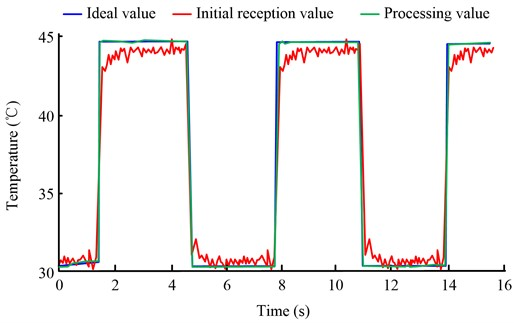Fig. 4Measurement error analysis of on-line measurement system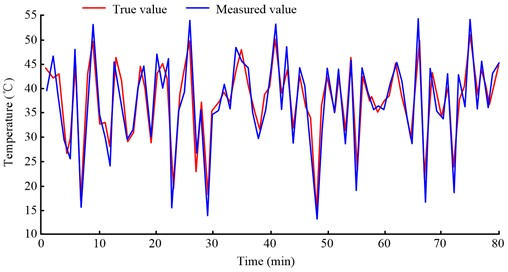Secondly, to specifically analyze the measurement accuracy and measurement efficiency of the OTMS of CNC, the changes of measurement accuracy and measurement time of the OTMS are studied and analyzed, see Fig. 5. In Fig. 5, the measurement accuracy and measurement time of the temperature measurement system are studied and evaluated. During the change of measurement accuracy, with the continuous increase of the number of measurement samples, the measurement accuracy of the system continues to improve, and when the sample is 500, the measurement accuracy of the system rises to more than 90 %. Finally, after the sample is 1500, the measurement accuracy of the system stabilized at 95 %. From the measurement time curve of the system, the measurement time of the system is decreasing with the increasing number of samples. When the sample is 500, the measurement time of the system is reduced to 4.0 s, and when the sample is 1500, the measurement time of the system is reduced to 2.0 s. The above results show that the OTMS proposed in the study has a high measurement accuracy, and its measurement time can be reduced continuously in long-term calculation, with high efficiency and high accuracy.

Fig. 5Measurement accuracy and time analysis of on-line measurement system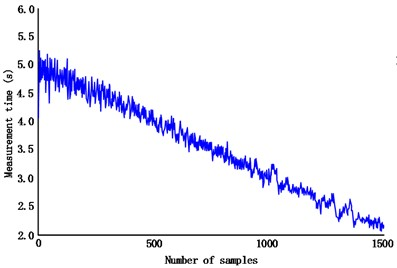a) Time variation of the temperature measurement system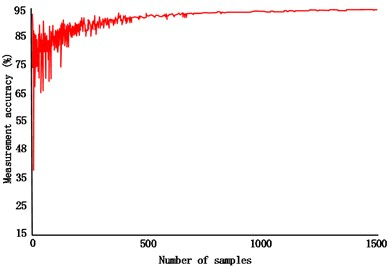b) Accuracy variation of the temperature measurement system

### 3.4. Performance analysis of adaptive TE model

The study utilizes BPNN to achieve temperature prediction and improves the traditional BPNN. In order to understand the effectiveness of the improved BPNN, its performance needs to be tested and analyzed. The experiment still takes the Leaderway V-450 machine tool as the research object, builds a dataset based on workpiece data from a certain manufacturing workshop, and divides it into a training set and a testing set to test the performance of the BPNN temperature prediction model. Firstly, evaluate the loss value changes of the improved BPNN during the training set testing process, as shown in Fig. 6. Fig. 6(a) shows the calculation results of various algorithms in the training set. The loss value of each algorithm in the training set decreases with the number of iterations. Among them, the loss value of the improved BPNN proposed in the study decreases more significantly in the training test of the training set. When the iterations is 20, the loss value of the improved BPNN decreases by less than 0.01, significantly lower than convolutional neural network (CNN) and artificial neural network (ANN). Fig. 6(b) shows the test results of each algorithm in the test set. The test results of the test set are similar to those of the training set, showing that the loss value of the improved BPNN decreases most rapidly and gradually decreases to the lowest point when the number of iterations is 30, and finally stabilizes below 0.01. The above results show that the study proposes an improved BPNN to achieve temperature prediction, and the test shows that the improved BPNN is feasible.

Fig. 6Loss value of BPNN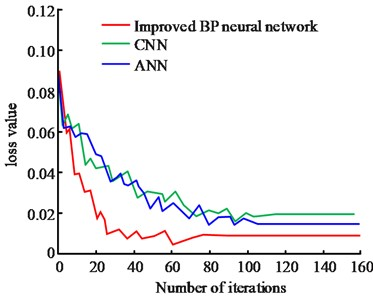a) Training set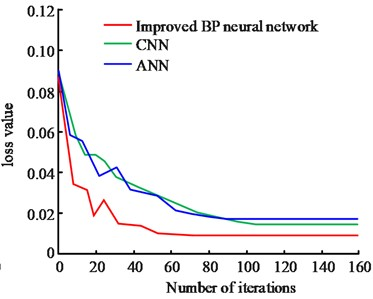b) Test set

Secondly, the specificity and sensitivity of the improved BPNN were analyzed using the receiver operating characteristic (ROC) curve, as shown in Fig. 7. The area under the ROC curve is an indicator for evaluating the quality of the model. In Fig. 7, the improved BPNN model clearly has better predictive performance, further proving the necessity and feasibility of the improved algorithm.

Fig. 7ROC curve of temperature prediction model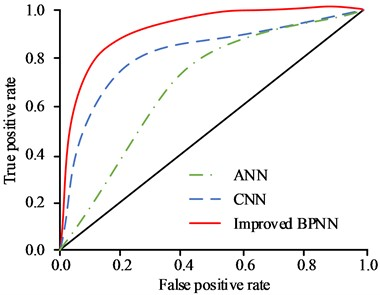Then, the effectiveness of the model is evaluated by analyzing the prediction residuals of the adaptive TE model in different data sets, see Fig. 8. In Fig. 8, the difference of prediction residuals of different algorithms on the $x$ and $y$ axes is compared. Fig. 8(a) shows the residual prediction on the $x$-axis and the comparison results of various algorithms. The prediction residual of each algorithm is all within 6×10-3 mm, the prediction residual of CNN algorithm is the largest, with the highest value reaching 5×10-3 mm, followed by the ANN algorithm, whose maximum residual error is only 3.1×10-3 mm, the minimum value is shown in the improved BPNN, and the maximum residual value is only 1.3×10-3 mm, significantly lower than other prediction algorithms. Fig. 8(b) shows the predicted residual value on y-axis. It can be found that the predicted residual value of ANN algorithm is still the largest, and the maximum value exceeds 6×10-3 mm, reaching 6.2×10-3 mm, followed by CNN algorithm, with the maximum predicted residual value reaching 3.2×10-3 mm. However, the maximum predictive residual value of the improved BPNN is only 1.1×10-3 mm, significantly lower than the other two prediction algorithms. The improved BPNN can significantly reduce the temperature prediction error, that is, improve its prediction accuracy, and effectively reduce the error of CNC processing.

Fig. 8Prediction error analysis of BPNN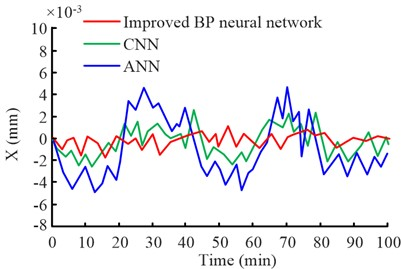a)$x$-axis residual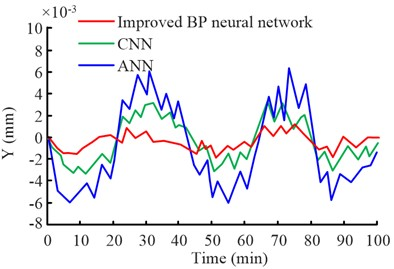b)$y$-axis residual

### 3.5. TE compensation effect of CNC

In the analysis of the TE compensation effect of CNC, the research first collects the position coordinates of CNC, including the original position of the work piece in the ideal state and the output position coordinates in the actual processing. First, plot the collected position coordinates, see Fig. 9. In Fig. 9, the variation of machining error of the CNC for opening the TE compensation function is analyzed. Fig. 9(a) shows the comparison results between the original position and the output position coordinates. The difference between the output coordinates of the CNC to enable TE compensation and the original coordinates is obvious, which means that under the influence of temperature, the machining accuracy of the CNC has decreased. Fig. 9(b) shows the error change during work piece processing. It can be seen from the change of processing error value that the maximum value of current CNC processing error exceeds 6×10-3 mm, and the average error reaches 3.8×10-3 mm. In addition, it can be seen from the variation of the repetitive error value of CNC processing that the research has a high consistency in error calculation, that is, the CNC error calculated by the research is feasible. The above results show that the errors produced by CNC processing are relatively obvious before TE compensation is introduced.

Fig. 9Machining error of CNC before TE compensation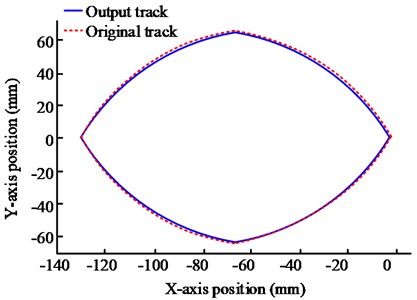a) Comparison of input track and output track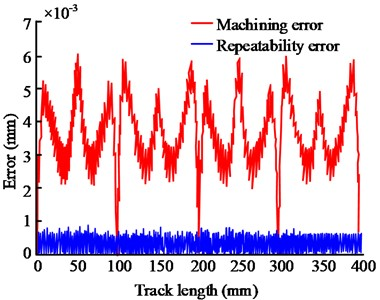b) Error and repeatability error

Fig. 10Comparison of optimal contour error before and after compensation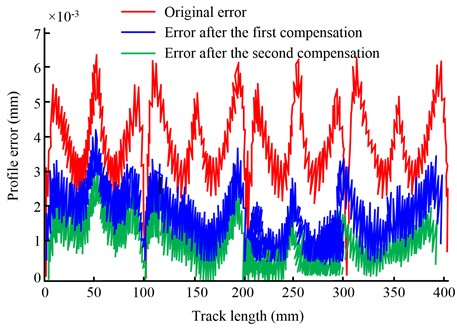After analyzing the errors generated during the processing of the CNC, the adaptive TECM is used to optimize the processing accuracy of the CNC, and the changes in the processing error of the CNC work piece shown in multiple compensations are compared, see Fig. 10. Fig. 10 shows that the study evaluated the change of work piece processing error after the adaptive TECM was processed twice. In the curve comparison in Fig. 10, the maximum initial error of work piece processing exceeds 6×10-3 mm. After the first error compensation, the error value of CNC work piece processing is reduced to below 4×10-3 mm, and it can be seen from the curve change that the minimum error at this time is reduced to 0.62×10-3 mm. After the second TE compensation, the machining error of CNC work piece is further reduced, and the maximum value is only 3.08×10-3 mm, and the minimum error is reduced to 0.01×10-3 mm. Compared with the difference of each curve, the machining error of CNC work piece after adaptive TE compensation is continuously reduced, and the contour error is obviously reduced after the second contour error compensation. From the whole curve, the profile error is mostly within 0.02×10-3 mm after the second compensation, and the repetition effect of contour error is good. The above results show that the improved TECM proposed in the study is feasible, and can significantly reduce the error of work piece processing, and improve the operating efficiency of CNC.

## 4. Conclusions

During the use of CNC, the TE caused by the temperature of the CNC seriously affects the machining accuracy of the work piece, which makes it difficult to improve the machining efficiency of the work piece and increases the workshop production cost. To reduce the influence of TE of CNC and realize the intellectualization of CNC, an improved adaptive TECM is proposed, which includes an OTMS and a temperature prediction model. To verify the application effect of the TECM, experimental tests are carried out on each functional part of the model. The results show that the proposed temperature online measurement system has a maximum accuracy of over 95 % and a measurement time of only 2 s, with high measurement accuracy and fast measurement speed. In the performance evaluation of the temperature prediction model, it was shown that the improved BPNN prediction algorithm proposed in the study predicted a loss value of less than 0.01, and in the error comparison of multiple algorithms, its maximum residual was only 1.3×10-3 mm, significantly lower than other prediction algorithms, indicating the feasibility of applying this algorithm to thermal error compensation models. Finally, in the performance analysis of the thermal error compensation model, it was shown that the proposed thermal error compensation model can continuously reduce the machining error of the workpiece during work. As the thermal error compensation progresses, the compensation model reduces the contour error of the workpiece machining to 0.02 after the second compensation × within 10-3 mm, the maximum contour error of the workpiece machining has decreased by about 50.32 % compared to the initial value. Overall, the thermal error compensation model proposed in the study can achieve adaptive error compensation for CNC machine tool processing, and can help achieve higher accuracy and efficiency in machine tool processing, which has a significant impact on improving workshop work efficiency. However, in the construction of the compensation model, the scalability of the proposed model needs to be further improved. Therefore, in the follow-up work, the amount of data collection will be expanded to improve the applicability of the model.

06 February 2023
Accepted
15 May 2023
Published
18 August 2023
SUBJECTS
System dynamics in manufacturing system modeling
Keywords
TE compensation
numerical control machine
BPNN
intelligence
Acknowledgements

The authors have not disclosed any funding.

Data Availability

The datasets generated during and/or analyzed during the current study are available from the corresponding author on reasonable request.

Author Contributions

Hui Shen: methodology; writing – original draft preparation; writing – review and editing. Liu Yang: data curation; formal analysis.

Conflict of interest

The authors declare that they have no conflict of interest.